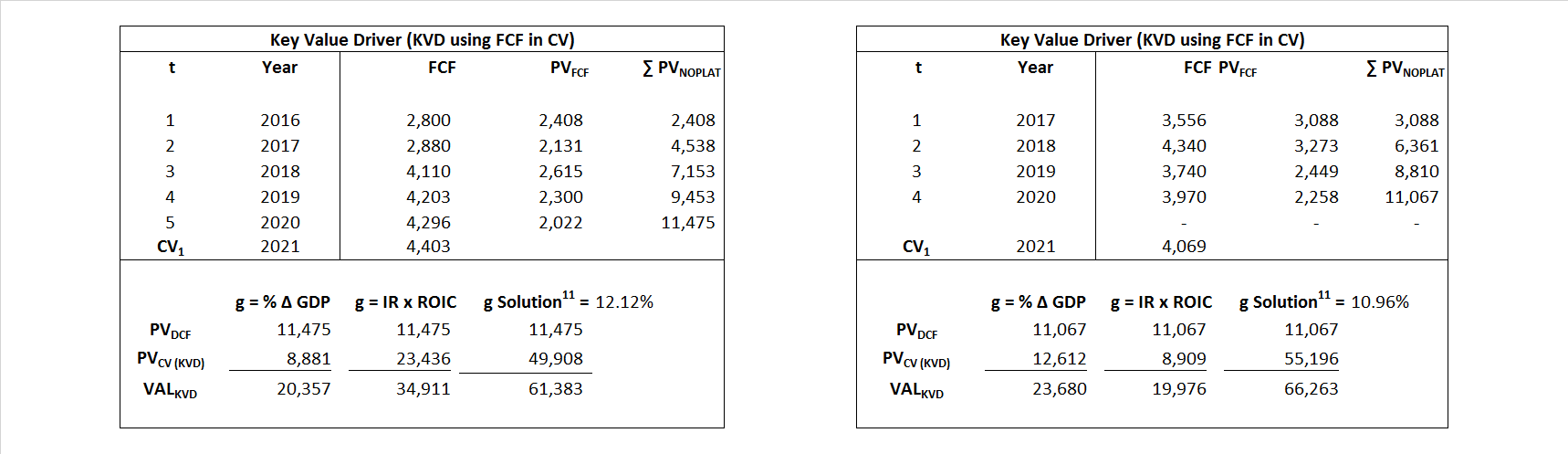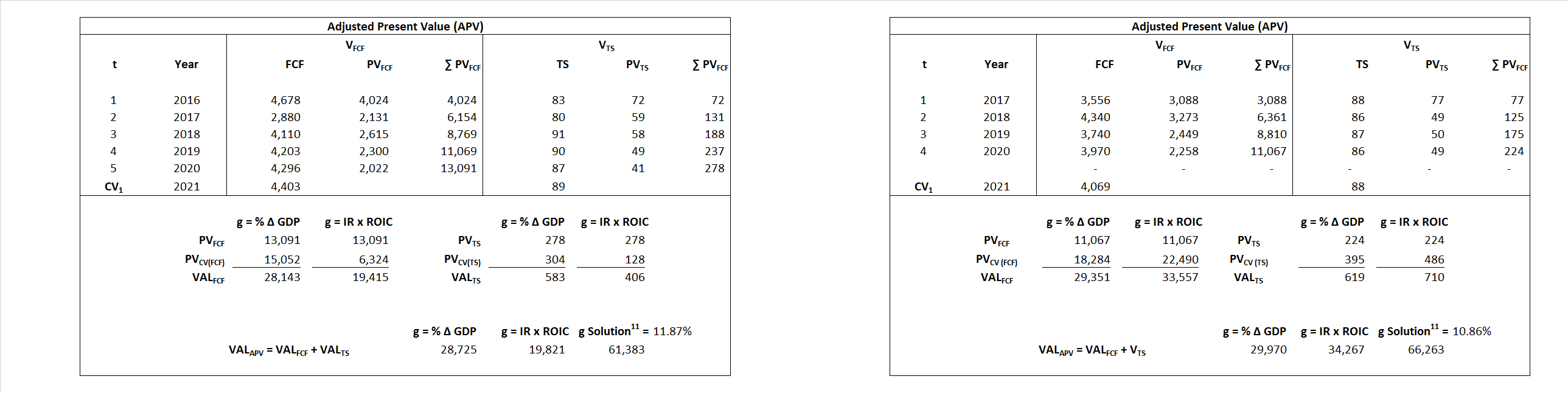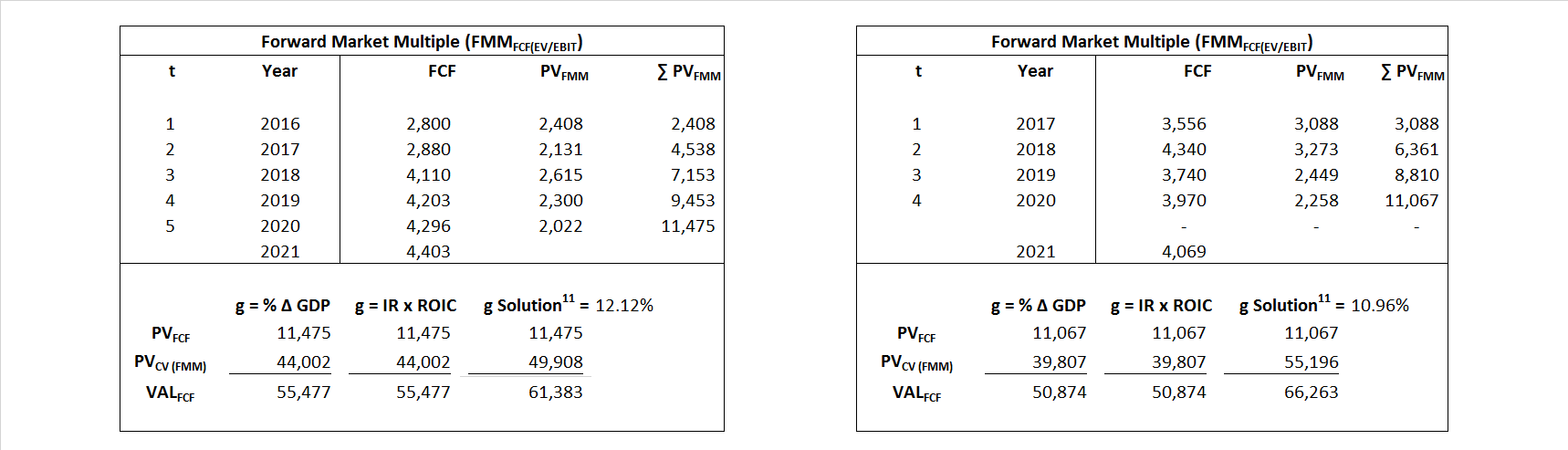# Du Pont

## Analyst Listing

The following analysts provide coverage for the subject firm as of May 2016:

 Broker Analyst Analyst Email Cowen & Company Colby Synesael colby.synesael@cowen.com Burke and Quick Partners, LLC Frederick W. Moran fmoran@bqpartners.com RBC Capital Markets Jonathan Atkin jonathan.atkin@rbccm.com Jefferies Jonathan Petersen jpetersen@jefferies.com Evercore ISI Jonathan Schildkraut schildkraut@evercoreisi.com Stifel Nicolaus Matthew Heinz heinzm@stifel.com

## Primary Input Data## Derived Input Data

### Equational Form

Net Operating Profit Less Adjusted Taxes NOPLAT  1,879  2,504$NOPLAT\, =\, EBIT\, x\, (1 \,-\, Avg \,\,Tax\,\, Rate\,\, on\,\, EBIT)$
Free Cash Flow FCF  687  2,281$FCF\,=NOPLAT\,+\,Non-Cash\,Expenses-\Delta NWC\,-\,NCS$
Tax Shield TS  87  85$TS\,=\,Interest\,\,Paid\,\,x\,\, Avg \,\,Tax\,\,Rate\,\, on\,\, Pre-Tax\,\, Income$
Invested Capital IC  30,813  31,067$IC\,=\,Fixed\,\,Operating\,\,Assets\,\,+\,\,Net\,\, Working\,\, Capital$
Return on Invested Capital ROIC 6.10% 8.06%$ROIC\,=\,\frac { NOPLAT }{ IC }$
Net Investment NetInv  (5,085)  1,512$NetInv\,=\,{ {IC}_{1}}-{{IC}_{0}}+Depreciation$
Investment Rate IR -270.60% 60.39%$IR\,=\,\frac {NetInv}{NOPLAT}$
Weighted Average Cost of Capital WACCMarket 16.27% 15.16%$WACC\,=\,\frac { E }{ V } { R }_{ E }\,+\,\frac { P }{ V } { R }_{ P }\,+\,\frac { D }{ V } { R }_{ D }\left( 1- Avg\,\, Tax\,\,Rate\,\,on\,\,Pre-Tax\,\,Income \right)$
WACCBook  8.11% 7.02%
Enterprise value EVMarket  61,383  66,263$EV\,=\,Market\,\,Cap\,\,Equity\,+\,\,Long\,\,Term\,\,Debt\,-\,Cash$
EVBook  59,468  65,484
EV/EBIT Multiple$\frac{EV_{Market}}{EBIT}$  21.23  17.20$EV/EBIT\,=\,\frac { EV}{ EBIT}$
Long-Run Growth g = IR x ROIC
-16.50%   4.87% Long-run growth rates of the income variable  are used in the Continuing Value portion of the valuation models.
g = %$\Delta$ GDP    2.50%   2.50%

## Valuation Model Outcomes

The outcomes presented in this study are the result of original input data, derived data, and synthesized inputs and, depending on the equational form of any particular valuation model, may result in irrelevant or implausible results.  For example, in the event WACC < g, the value of this term, often found in the denominator of an equation’s continuation value term, will be expressly negative and may result in a negative overall valuation for the firm.  In the event of a WACC < g relation, the model form as applied to the subject firm offers an irrelevant outcome.

### Equational form

Key Value Driver (NOPLAT) KVD (NOPLAT)${ Value }_{ DCF/KVD }=\sum { \frac { NOPLAT_{ t } }{ { \left( 1+WACC \right) }^{ t } } +\frac { \frac { { NOPLAT }_{ 1 }\left( 1-\frac { g }{ ROIC } \right) }{ WACC-g } }{ { \left( 1+WACC \right) }^{ t } } }$Key Value Driver (FCF) KVD (FCF)${ Value }_{ DCF/KVD }=\sum { \frac { FCF_{ t } }{ { \left( 1+WACC \right) }^{ t } } +\frac { \frac { { NOPLAT }_{ 1 }\left( 1-\frac { g }{ ROIC } \right) }{ WACC-g } }{ { \left( 1+WACC \right) }^{ t } } }$Free Cash Flow FCF${ Value }_{ DCF/FCF }=\sum { \frac { FCF_{ t } }{ { \left( 1+WACC \right) }^{ t } } +\frac { \frac { { FCF }_{ 1 }}{ WACC-g } }{ { \left( 1+WACC \right) }^{ t } } }$Economic Profit ECON π${ Value }_{ { ECON\pi } }= I{ C }_{ 0 }+\sum { \frac { { IC }_{ t-1 }(ROI{ C }_{t}-WAC{C}_{t}) }{ { \left( 1+WACC \right) }^{ t } }+ \frac {\frac { I{C}_{0}\ x\ (ROI{C}_{1}\ -\ WAC{C}_{1}) }{ WACC-g } }{ { \left( 1+WACC \right) }^{ t } } }$Adjusted Present Value APV${ Value }_{ APV }=\sum { \frac { FCF_{ t } }{ { \left( 1+{ k }_{ u } \right) }^{ t } } +\frac { \frac { { FCF }_{ 1 }}{ { k }_{ u }-g } }{ { \left( 1+{ k }_{ u } \right) }^{ t } } } +\sum { \frac { { TS }_{ t } }{ { \left( 1+{ k }_{ tax } \right) }^{ t } } +\frac { \frac { { TS }_{ 1 }}{ { k }_{ tax }-g } }{ { \left( 1+{ k }_{ tax } \right) }^{ t } } }$Forward Market Multiple FMM${ Value }_{ DCF/FMM}=\sum { \frac { FCF_{ t } }{ { \left( 1+WACC \right) }^{ t } } +\frac { { EBIT }_{ 1 }\,{x}\,{FMM}}{ { \left( 1+WACC \right) }^{ t } } }{\,\,\,; \,\,FMM\,=\,\frac{{EV}_{t=0}}{{EBIT}_{t=0}}}$